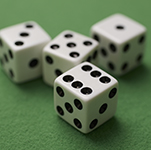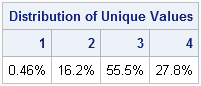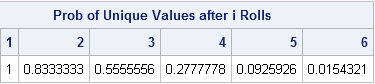Tossing dice is a simple and familiar process, yet it can illustrate deep and counterintuitive aspects of random numbers. For example, if you toss four identical six-sided dice, what is the probability that the faces are all distinct, as shown to the left? Many people would guess that the probability is fairly high, and that the chance of getting duplicates values (pairs, three of a kind, or four of a kind) is low. In an informal survey of some of my nonstatistical friends, more than half guessed 50%–60% of tosses would result in four distinct values.

Those guesses are about twice as high as reality. Most rolls will contain duplicates. In order to get distinct values (no duplicates), the first die can show any of six faces, the second die can show any of five faces, and so on. The total number of possible rolls for four dice is 64. Consequently, the probability of rolling distinct values with four dice is (6*5*4*3) / 64 = 0.277 or about 28%.

Equivalently, 72% of the tosses result in one or more duplicate values. If you feel more comfortable with simulation than with combinatorics, the following statements simulate one million rolls of four dice. I count the number of unique values for each roll and tabulate the results:

```proc iml; /* Let X = number of unique values in 6 rolls of a six-sided die. Simulate distribution of X. */ N = 1e6; r = j(N, 6); call randseed(12345); call randgen(r, "Uniform"); /* ~ U(0,1) */ r = ceil(6*r); /* ~ U({1,2,3,4,5,6}) */ x = countunique(r, "row"); call tabulate(vals, freq, x); print (freq/N)[label="Distribution of Unique Values" format=percent7.2 colname=(char(vals))];```The simulation shows that a small percentage of the rolls (0.46%) result in one unique value (all four dice show the same value). About 16% of the rolls result in two unique values (the dice show two pairs or three of a kind). More than 55% of the time you will see three unique values (one pair). And, as shown theoretically, only about 28% of the rolls result in four unique values (no pairs).

Many statistical programmers have seen the famous "birthday matching problem," which states that the probability of duplicate birthdays among a small group of people is much higher than most people would guess. Among 23 people, the probability of a duplicate birthday is more than 50%. Among 40 people, the probability of two people sharing a birthday is about 90%.

You can compute similar probabilities for dice. The computations are easier if you compute the probabilities of "no duplicates." Suppose that you toss a die N times. Let QN be the probability that there are no duplicates among the N rolls. As I have explained previously, the following SAS/IML statements compute the probabilities QN for N=1,...,6:

```M = 6; /* max number of unique values (faces) */ i = 1:6; /* enumerate trials (tosses) */ iQ = 1 - (i-1)/M; /* individual probabilities */ Q = cuprod(iQ); /* compute probability of "no match" after the i_th trial */ print Q[label="Probability of Unique Values after i Rolls" colname=(char(i))];```As you can see, the probability of unique values after N rolls decreases quickly. Equivalently, the probability of duplicates increases quickly. The probability of seeing a duplicate value is 45% after three rolls, 72% after four rolls, and a whopping 91% after five rolls.

Why should you care about any of this? Well, as I wrote at the beginning of this post, dice illustrate aspects of random numbers. This post was motivated by the fact that a SAS customer reported to Technical Support that he was getting some duplicate values when he used the RAND function to generate a million random uniform values on the interval [0,1]. He wanted to know if this result indicates a bug in the RAND function. No. The dice example in this post teaches two lessons:

• In a long stream of uniformly distributed random numbers, you should expect to see duplicate values. If you do not see duplicate values, that indicates that the stream is not very random.
• As the birthday matching problem shows, duplicates occur in samples that are much smaller than you might intuitively expect.

In my next post I will discuss numbers that are generated by the SAS RAND function. I will show that you should expect to see duplicate values in a large sample of uniformly distributed random values. But how large? Do you need to generate a million values? Nope. You can expect duplicate values in much smaller samples. Stay tuned.

Share